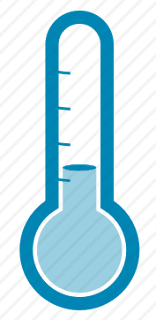Temperature Converter | SJB -->

## Pages

### Temperature Converter

There are three temperature scales in use today, Fahrenheit, Celsius, and Kelvin. The Fahrenheit scale was developed by the German physicist Daniel Gabriel Fahrenheit (1686-1736). He originally took as the zero of his scale the temperature of an equal ice-salt mixture and selected the values of 30 and 90 for the freezing point of water and normal body temperature, respectively; these later were revised to 32 and 96, but the final scale required an adjustment to 98.6 for the latter value. Fahrenheit temperature scale is a scale based on 32 for the freezing point of water and 212 for the boiling point of water, the interval between the two being divided into 180 parts. The conversion formula for a temperature that is expressed on the Celsius(C) scale to its Fahrenheit(F) representation is:

F = (9 / 5) * (C + 32)

Celsius temperature scale also called Centigrade temperature scale, was developed by Swedish astronomer Andres Celsius (1701-1744), in 1742. It is sometimes called the centigrade scale because of the 100-degree interval between the defined points. In this scale, water freezes at 0 degrees and boils at 100 degrees. The Celsius scale is in general use wherever metric units have become accepted. The centigrade to Fahrenheit conversion formula is:

C = (5 / 9) * (F - 32)

For an exact conversion (Fahrenheit to Celsius / Celsius to Fahrenheit) the following formulas can be applied:
Fahrenheit to Celsius: (°F − 32) ÷ 1.8 = °C
Celsius to Fahrenheit: (°C * 1.8) + 32 = °FTemperature in Celsius:
Temperature in Fahrenheit:
Temperature in Kelvin:

An approximate conversion between degrees Celsius and degrees Fahrenheit is as follows:
Fahrenheit to Celsius: Subtract 30 and halve the resulting number.
Celsius to Fahrenheit: Double the number and add 30.

Kelvin temperature scale is the base unit of thermodynamic temperature measurement in the International System (SI) of measurement. It is defined as 1/273.16 of the triple point (equilibrium among the solid, liquid, and gaseous phases) of pure water. The kelvin (symbol K without the degree sign) is also the fundamental unit of the Kelvin scale, an absolute temperature scale named for the Scottish mathematician and physicist Sir William Thomson, Baron Kelvin (1824 - 1907). Such a scale has as its zero point absolute zero, the theoretical temperature at which the molecules of a substance have the lowest energy. Many physical laws and formulas can be expressed more simply when an absolute temperature scale is used; accordingly, the Kelvin scale has been adopted as the international standard for scientific temperature measurement. The Kelvin scale is related to the Celsius scale. The difference between the freezing and boiling points of water is 100 degrees in each so that the Kelvin has the same magnitude as the degree Celsius.

`var numposts = 5;var showpostthumbnails = true;var displaymore = true;var displayseparator = false;var showcommentnum = false;var showpostdate = false;var showpostsummary = true;var numchars = 40;`
Below is the little script that used for the converter_
`123456789101112`function` `CelConvert() {``    ``TempVS.inFah.value = (TempVS.inCel.value * 9 / 5) + 32 + (``' \xB0F'``);``    ``TempVS.inKel.value = TempVS.inCel.value * 1 + 273.15 + (``' K'``);``}``function` `FahConvert() {``    ``TempVS.inCel.value = (TempVS.inFah.value - 32) * 5 / 9 + (``' \xB0C'``);``    ``TempVS.inKel.value = ((TempVS.inFah.value - 32) * 5 / 9) + 273.15 + (``' K'``);``}``function` `KelConvert() {``    ``TempVS.inCel.value = TempVS.inKel.value - 273.15 + (``' \xB0C'``);``    ``TempVS.inFah.value = ((TempVS.inKel.value - 273.15) * 9 / 5) + 32 + (``' \xB0F'``);``}``